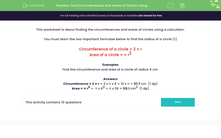# Find the Circumference and Area of a Circle

In this worksheet, students will find the circumferences and areas of circles given their radii. They use a calculator to work out their answers.Key stage:  KS 3

Curriculum topic:   Number

Curriculum subtopic:   Use Calculators/Technology for Accuracy

Popular topics:   Area worksheets

Difficulty level:#### Worksheet Overview

This activity is about finding the circumferences and areas of circles using a calculator.

We need to learn the two important formulae below using the radius of a circle (r)

Circumference of a circle = 2 π r

Area of a circle = π r2

Examples

Find the circumference and area of a circle of radius 6 cm.

π is usually taken to be 3.142, but your calculator should have the π button on it which will make your calculations more accurate. Once you've done the first part of the calculation, just tap in x π and then = and you should get the correct answer. Test it out with the examples below!

Circumference = 2 π r = 2 x π x 6 = 12 x π = 37.7 cm  (1 dp)

Area = π r2 =  π x 62 = π x 36 = 113.1 cm2  (1 dp)

On to the questions now!

### What is EdPlace?

We're your National Curriculum aligned online education content provider helping each child succeed in English, maths and science from year 1 to GCSE. With an EdPlace account you’ll be able to track and measure progress, helping each child achieve their best. We build confidence and attainment by personalising each child’s learning at a level that suits them.

Get started